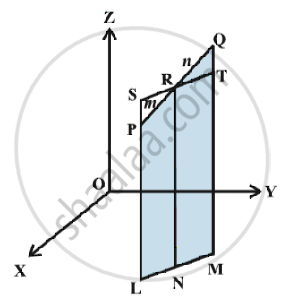# Three - Dimensional Geometry - Section Formula

## Notes

Let the two given points be P(x_1, y_1, z_1) and Q (x_2, y_2, z_2). Let the point R (x, y, z) divide PQ in the given ratio m : n internally. Draw PL, QM and RN perpendicular to the XY-plane. Obviously PL || RN || QM and feet of these perpendiculars lie in a XY-plane. The points L, M and N will lie on a line which is the intersection of the plane containing PL, RN and QM with the XY-plane. Through the point R draw a line ST parallel to the line LM. Line ST will intersect the line LP externally  at the point S and the line MQ at T, FigAlso note that quadrilaterals LNRS and NMTR are parallelograms. The triangles PSR and QTR are similar. Therefore,
m/n = (PR)/(QR) = (SP)/(QT) = (SL-PL)/(QM-TM) = (NR-PL)/(QM-NR) = (z-z_1)/(z_2-z)

This implies z = (mz_2+nz_1)/(m+n)

Hence, the coordinates of the point R which divides the line segment joining two points P (x_1, y_1, z_1) and Q (x_2, y_2, z_2) internally in the ratio m : n are

[(mx_2+nx_1)/(m+n) , (my_2+ny_1)/(m+n ), (mz_2 +nz_1)/(m+n)]

If the point R divides PQ externally in the ratio m : n, then its coordinates are obtained by replacing n by – n so that coordinates of point R will be

[(mx_2-nx_1)/(m+n) , (my_2-ny_1)/(m+n) , (mz_2 -nz_1)/(m+n)]

Case 1- Coordinates of the mid-point: In case R is the mid-point of PQ, then

m:n = 1:1 so that  x =(x_1-x_2)/2 ,y= (y_1+y_2)/2 and z=(z_1+z_2)/2
These are the coordinates of the mid point of the segment joining P (x_1, y_1, z_1) and Q (x_2, y_2, z_2).
Case 2-   The coordinates of the point R which divides PQ in the ratio k : 1 are obtained

by taking k = m/n which are as given below:

[(kx_2+x_1)/(1+k),(ky_2+y_1)/(1+k),(kz_2+z_1)/(1+k)]

If you would like to contribute notes or other learning material, please submit them using the button below.

### Shaalaa.com

Section formula [00:05:13]
S
0%